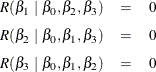# The Four Types of Estimable Functions

### Examples

Subsections:

#### A One-Way Classification Model

For the model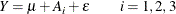the general form of estimable functions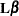is (from the previous example)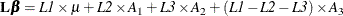Thus,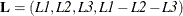Tests involving only the parameters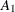,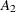, and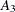must have an
of the form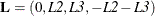Since thisfor the A parameters involves only two symbols, hypotheses with at most two degrees of freedom can be constructed. For example, letting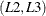be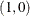and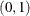, respectively, yields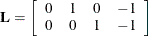The precedingcan be used to test the hypothesis that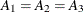. For this example, anywith two linearly independent rows with column 1 equal to zero produces the same sum of squares. For example, a joint test for linear and quadratic effects of A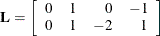gives the same SS. In fact, for anyof full row rank and any nonsingular matrix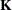of conformable dimensions,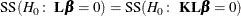#### A Three-Factor Main-Effects Model

Consider a three-factor main-effects model involving the CLASS variables A, B, and C, as shown in Table 15.1.

Table 15.1: Three-Factor Main-Effects Model

Obs

A

B

C

1

1

2

1

2

1

1

2

3

2

1

3

4

2

2

2

5

2

2

2

The general form of an estimable function is shown in Table 15.2.

Table 15.2: General Form of an Estimable Function for Three-Factor Main-Effects Model

Parameter

Coefficient(Intercept)

L1

A1

L2

A2

L1L2

B1

L4

B2

L1L4

C1

L6

C2

L1 + L2L4 – 2L6

C3

L2 + L4 + L6

Since only four symbols (L1, L2, L4, and L6) are involved, any testable hypothesis will have at most four degrees of freedom. If you form anmatrix with four linearly independent rows according to the preceding rules, then testing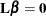is equivalent to testing that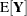is uniformly 0. Symbolically,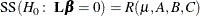In a main-effects model, the usual hypothesis of interest for a main effect is the equality of all the parameters. In this example, it is not possible to unambiguously test such a hypothesis because of confounding: any test for the equality of the parameters for any one of A, B, or C will necessarily involve the parameters for the other two effects. One way to proceed is to construct a maximum rank hypothesis (MRH) involving only the parameters of the main effect in question. This can be done using the general form of estimable functions. Note the following:

• To get an MRH involving only the parameters of A, the coefficients ofassociated with, B1, B2, C1, C2, and C3 must be equated to zero. Starting at the top of the general form, let L1 = 0, then L4 = 0, then L6 = 0. If C2 and C3 are not to be involved, then L2 must also be zero. Thus, A1A2 is not estimable; that is, the MRH involving only the A parameters has zero rank and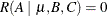.

• To obtain the MRH involving only the B parameters, let L1 = L2 = L6 = 0. But then to remove C2 and C3 from the comparison, L4 must also be set to 0. Thus, B1B2 is not estimable and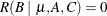.

• To obtain the MRH involving only the C parameters, let L1 = L2 = L4 =0. Thus, the MRH involving only C parameters is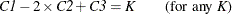or any multiple of the left-hand side equal to K. Furthermore,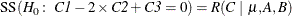#### A Multiple Regression Model

Suppose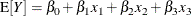where thematrix has full rank. The general form of estimable functions is as shown in Table 15.3.

Table 15.3: General Form of Estimable Functions for a Multiple Regression Model WhenMatrix Is of Full Rank

Parameter

Coefficient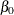L1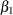L2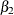L3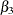L4

For example, to test the hypothesis that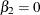, let L1 = L2 = L4 = 0 and let L3 = 1. Then SS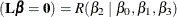. In this full-rank case, all parameters, as well as any linear combination of parameters, are estimable.

Suppose, however, that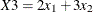. The general form of estimable functions is shown in Table 15.4.

Table 15.4: General Form of Estimable Functions for a Multiple Regression Model WhenMatrix Is Not of Full Rank

Parameter

CoefficientL1L2L3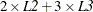For this example, it is possible to test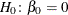. However,,, andare not jointly estimable; that is,